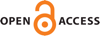#### Vol. 11, No. 1, 2021

 Download this articleFor screen For printingRecent Issues Volume 13, Issue 1 Volume 12, Issue 1 Volume 11, Issue 1 Volume 10, Issue 1 Volume 9, Issue 2 Volume 9, Issue 1 Volume 8, Issue 1 Volume 7, Issue 1 Volume 6, Issue 1 Volume 5, Issue 1 Volume 4, Issue 1 Volume 3, Issue 1 Volume 2, Issue 1 Volume 1, Issue 1The Journal About the Journal Editorial Board Submission Guidelines Submission Form Policies for Authors Ethics Statement ISSN: 1948-7916 Author Index To Appear Other MSP JournalsExteriorModules: a package for computing monomial modules over an exterior algebra

### Luca Amata and Marilena Crupi

Vol. 11 (2021), 71–81##### Abstract

Let $K$ be a field, $E$ the exterior algebra of a finite-dimensional $K$-vector space, and $F$ a finitely generated graded free $E$-module with homogeneous basis ${g}_{1},\dots ,{g}_{r}$ such that $deg\left({g}_{1}\right)\le deg\left({g}_{2}\right)\le \cdots \le deg\left({g}_{r}\right)$. We present a Macaulay2 package to manage some classes of monomial submodules of $F$. The package is an extension of our ExteriorIdeals package on monomial ideals (J. of Software for Alg. and Geom. 8:7 (2018), 71–79), and contains some algorithms for computing stable, strongly stable and lexicograhic $E$-submodules of $F$. This package also includes some methods to check whether a sequence of nonnegative integers is the Hilbert function of a graded $E$-module of the form $F∕M$, with $M$ a graded submodule of $F$. Moreover, if ${H}_{F∕M}$ is the Hilbert function of a graded $E$-module $F∕M$, some routines are able to compute the unique lexicograhic submodule $L$ of $F$ such that ${H}_{F∕M}={H}_{F∕L}$.

##### Keywords
exterior algebra, monomial modules, Hilbert functions, algorithms
##### Mathematical Subject Classification
Primary: 13A02, 15A75, 68W30
##### Supplementary material

Monomial modules over exterior algebras